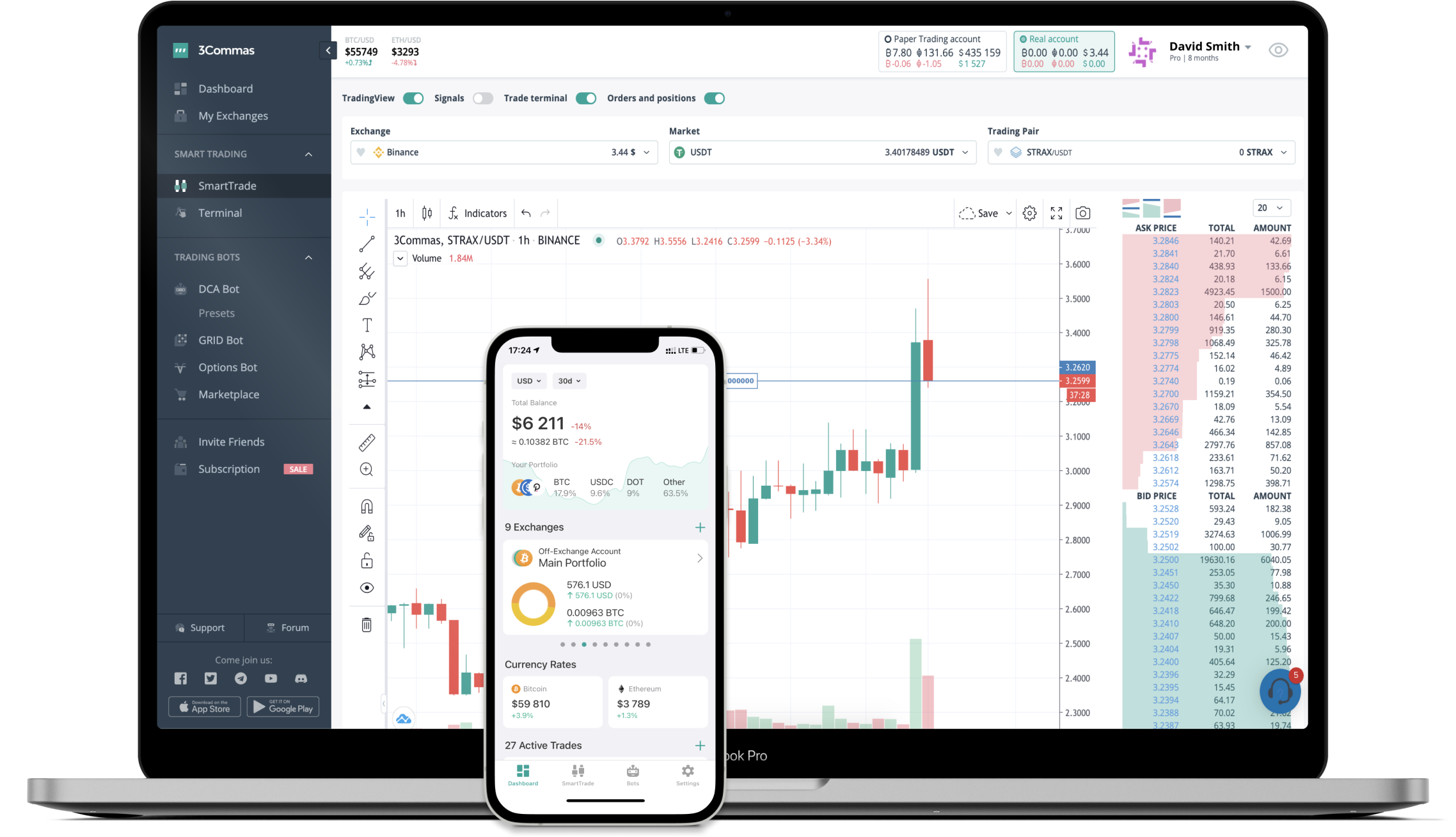# DELTA to CNY Converter

Delta Financial
PriceCNY 8.02+0.11%
VolumeCNY 6,210
How it work

The 3Commas currency calculator allows you to convert a currency from Delta Financial (DELTA) to Chinese Yuan (CNY) in just a few clicks at live exchange rates.

Simply enter the amount of Delta Financial you wish to convert to CNY and the conversion amount automatically populates. You can also use our Prices Calculator Table to calculate how much your currency is worth in other denominations, i.e. .1 DELTA, .5 DELTA, 1 DELTA, 5 DELTA, or even 10 DELTA.

## DELTA to CNY Exchange Calculator

The chart displays Delta Financial price changes in CNY.

The ultimate tools for crypto traders to maximize trading profits while minimizing risk and loss.## Latest DELTA to CNY price calculator

Delta Financial market price is updated every three minutes and is automatically displayed in CNY. Below are the most popular denominations to convert to CNY.

Delta Financial
Chinese Yuan
0.08
CNY
0.80
CNY
8.02
CNY
16.04
CNY
24.06
CNY
40.10
CNY
80.20
CNY
200.50
CNY
401.00
CNY
802.00
CNY
2005.00
CNY
4010.00
CNY
8020.00
CNY
20050.00
CNY
Chinese Yuan
Delta Financial
0.00124688
DELTA
0.01246883
DELTA
0.12468828
DELTA
0.24937656
DELTA
0.37406484
DELTA
0.62344140
DELTA
1.24688279
DELTA
3.11720698
DELTA
6.23441397
DELTA
12.46882793
DELTA
31.17206983
DELTA
62.34413965
DELTA
124.68827930
DELTA
311.72069825
DELTA

## FAQ

### How much is 1 Delta Financial in Chinese Yuan?

Delta Financial price in CNY is constantly changing.

At this moment, 1 Delta Financial equals 8.02 CNY

### How do I calculate the conversion from DELTA to CNY?

The 3Commas Delta Financial Calculator allows you to easily calculate the conversion price of DELTA to CNY by simply entering the amount of Delta Financial in the corresponding field and will automatically convert the value in Chinese Yuan (CNY).

You can also use our Delta Financial price table above to check the latest Delta Financial price in major fiat and crypto currencies.

### How can I convert Delta Financial to CNY?

The most common way of converting DELTA to CNY is by using a Crypto Exchange or a P2P (person-to-person) exchange platform like LocalBitcoins, etc.